# Derived set

(diff) ← Older revision | Latest revision (diff) | Newer revision → (diff)

The collection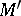of all limit points of a setin a topological space (cf. Limit point of a set). A setthat coincides with its derived set is called perfect.

This process can be iterated.

In general one defines, for an ordinal number, the-th derived set of,, as follows:,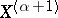is the derived set of, and ifis a limit ordinal then.

One then shows that there is a first ordinal number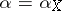such that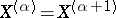. If, thenis called scattered; if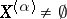, thenis called the perfect kernel of.

In this way one can prove the Cantor–Bendixson theorem: Ifis a subspace of the real line, then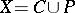, witha countable set,a perfect set and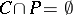.

For this reason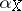is sometimes called the Cantor–Bendixson height of. Perfect spaces are sometimes called dense-in-itself.

How to Cite This Entry:
Derived set. Encyclopedia of Mathematics. URL: http://encyclopediaofmath.org/index.php?title=Derived_set&oldid=11226
This article was adapted from an original article by M.I. Voitsekhovskii (originator), which appeared in Encyclopedia of Mathematics - ISBN 1402006098. See original article